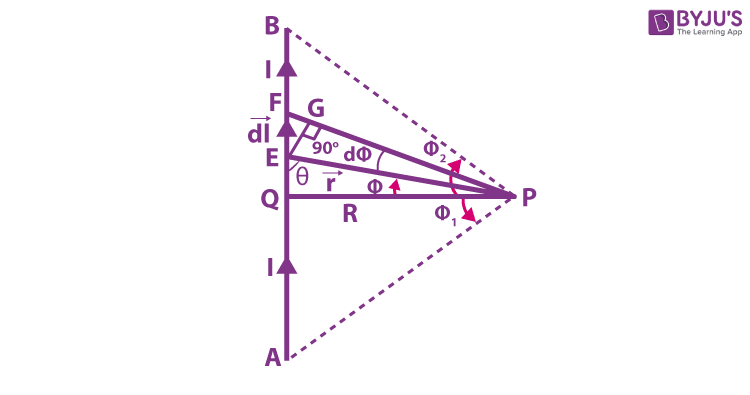# Magnetic field Intensity Due To A Straight Current-Carrying Conductor Of Finite Length

We have seen and played with magnets since childhood and have witnessed magnets used in many devices like AC, refrigerators, TV, earphones and also as door catchers. Magnets are used in various applications in our day to day life. Magnet is a material that has the capacity to create magnetic fields. The area around a magnet where the magnetic force can be felt is known as the magnetic field. This magnetic field cannot be seen and is the notable property of a magnet.

It is a vector quantity that defines the area of influence of the magnet. In this article, let us learn about magnetic field intensity due to a straight current-carrying conductor of finite length.

## Magnetic Field Intensity Due To A Straight Current-Carrying Conductor Of Finite Length

As we know, current-carrying conductors experience magnetic fields. Any mass will produce a gravitational field and can also interact with that field. Charge produces an electric field and also interacts with that field. Since moving charge interacts with a magnetic field, we might expect that it also creates that field.

Consider a straight conductor AB carrying a current (I), and magnetic field intensity is to be determined at point P.Refer to the above image. According to Biot-Savart law, the magnetic field at P is given by

Let AB be the conductor through which current I flows. Consider a point P, placed at a certain distance from the midpoint of the conductor. Consider the small current-carrying element dl placed at point c. It is at a distance r from point p. l be the distance between the centre of the coil and dl is the length. Using the biot-savart law, the magnetic field at point P due to current carrying element dl is

$$\bar{dB} = \frac{\mu _{0}}{4\pi }. \frac{I\bar{dl}.\bar{r}}{r^{3}}$$

Since the angle between angle between I dl and r is (180 degrees) hence,

$$\bar{dB} = \frac{\mu _{0}}{4\pi }. \frac{I\bar{dl}.sin (180 – \Theta )}{r^{2}}$$

$$\bar{dB} = \frac{\mu _{0}}{4\pi }. \frac{I{dl}.sin\theta }{r^{2}}$$ ——————- (1)

In this case $$EG = EF sin\theta$$

= $$dl sin\theta$$

$$EG = EP sin d\phi = rsin d\phi$$

= $$rd\phi$$

$$dl sin\theta = rd\phi$$ ——————- (2)

From equation (1)

$${dB} = \frac{\mu _{0}}{4\pi }. \frac{I\bar{d\phi}}{r}$$ ——————- (3)

from $$\Delta EQP$$

$$r = \frac{R}{cos\phi }$$

Hence

$${dB} = \frac{\mu _{0}}{4\pi }. \frac{Icos\phi d\phi }{R}$$ ———————–(4)

Then the total magnetic field at point P due to the complete length of the conductor is

$${B} = \int_{-\phi 1}^{\phi 2}\frac{\mu _{0}}{4\pi }. \frac{I}{R} cos\phi d\phi$$

From $$-\phi1$$ to $$\phi 2$$

{B} = \frac{\mu _{0}}{4\pi }. \frac{I}{R} \sin\phi

Hence

$${B} = \frac{\mu _{0}}{4\pi }. \frac{I}{R} \sin\phi$$ ———————–(5)

For any conductor of infinite length

$$\phi 1 = \phi 2 = 90^{0}$$

$${B} = \frac{\mu _{0}}{4\pi }. \frac{2I}{R}$$

$${B} = \frac{\mu _{0}}{2\pi }. \frac{I}{R}$$

$${B} = \frac{\mu _{0}}{2\pi }. \frac{I}{R} N A^{-1}m^{-1}$$

The direction of magnetic field due to a current carrying conductor can be obtained by using laws like

(i) Right hand palm rule no 1

(ii) Right hand thumb rule

(iii) Maxwell Right-hand screw Rule

Stay tuned with BYJU’S for more such interesting derivations in physics, chemistry and maths in an engaging way with video explanations.

## Frequently Asked Questions on Magnetic Field

Tesla.

### 2. Magnetic field is represented using which alphabet?

Magnetic field is represented using the letter B.

### 3. If the wire in the form of a loop forms a full circle of radius R with current I. What is the magnitude of the magnetic field at the center?

$${B} = \frac{\mu _{0}I}{R }$$

### 4. Electric field is a scalar or vector quantity?

The electric field is a vector quantity.

### 5. What is an electrical conductor?

Electrical conductor is an object or type of material that allows the flow of charge through it.